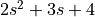# control.tf¶

control.tf(num, den[, dt])[source]

Create a transfer function system. Can create MIMO systems.

The function accepts either 1, 2, or 3 parameters:

`tf(sys)`

Convert a linear system into transfer function form. Always creates a new system, even if sys is already a TransferFunction object.

`tf(num, den)`

Create a transfer function system from its numerator and denominator polynomial coefficients.

If num and den are 1D array_like objects, the function creates a SISO system.

To create a MIMO system, num and den need to be 2D nested lists of array_like objects. (A 3 dimensional data structure in total.) (For details see note below.)

`tf(num, den, dt)`

Create a discrete time transfer function system; dt can either be a positive number indicating the sampling time or ‘True’ if no specific timebase is given.

`tf('s')` or `tf('z')`

Create a transfer function representing the differential operator (‘s’) or delay operator (‘z’).

Parameters
• sys (LTI (StateSpace or TransferFunction)) – A linear system

• num (array_like, or list of list of array_like) – Polynomial coefficients of the numerator

• den (array_like, or list of list of array_like) – Polynomial coefficients of the denominator

• display_format (None, 'poly' or 'zpk') – Set the display format used in printing the TransferFunction object. Default behavior is polynomial display and can be changed by changing config.defaults[‘xferfcn.display_format’]..

• inputs (str, or list of str, optional) – List of strings that name the individual signals of the transformed system. If not given, the inputs and outputs are the same as the original system.

• outputs (str, or list of str, optional) – List of strings that name the individual signals of the transformed system. If not given, the inputs and outputs are the same as the original system.

• name (string, optional) – System name. If unspecified, a generic name <sys[id]> is generated with a unique integer id.

Returns

out – The new linear system

Return type

`TransferFunction`

Raises
• ValueError – if num and den have invalid or unequal dimensions

• TypeError – if num or den are of incorrect type

Notes

`num[i][j]` contains the polynomial coefficients of the numerator for the transfer function from the (j+1)st input to the (i+1)st output. `den[i][j]` works the same way.

The list `[2, 3, 4]` denotes the polynomial.

The special forms `tf('s')` and `tf('z')` can be used to create transfer functions for differentiation and unit delays.

Examples

```>>> # Create a MIMO transfer function object
>>> # The transfer function from the 2nd input to the 1st output is
>>> # (3s + 4) / (6s^2 + 5s + 4).
>>> num = [[[1., 2.], [3., 4.]], [[5., 6.], [7., 8.]]]
>>> den = [[[9., 8., 7.], [6., 5., 4.]], [[3., 2., 1.], [-1., -2., -3.]]]
>>> sys1 = ct.tf(num, den)
```
```>>> # Create a variable 's' to allow algebra operations for SISO systems
>>> s = ct.tf('s')
>>> G  = (s + 1)/(s**2 + 2*s + 1)
```
```>>> # Convert a StateSpace to a TransferFunction object.
>>> sys_ss = ct.ss("1. -2; 3. -4", "5.; 7", "6. 8", "9.")
>>> sys2 = ct.tf(sys1)
```